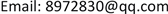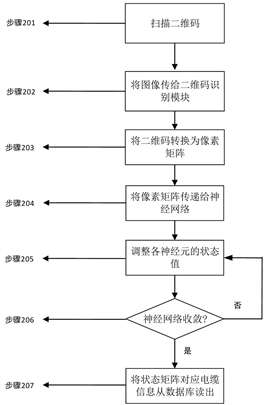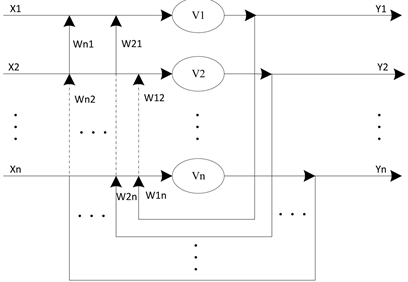﻿ 基于神经网络的二维码识别算法 Two-Dimensional Code Recognition Algorithm Based on Neural Network

Computer Science and Application
Vol. 08  No. 10 ( 2018 ), Article ID: 27237 , 6 pages
10.12677/CSA.2018.810169

Two-Dimensional Code Recognition Algorithm Based on Neural Network

Tongqing Xu, Haoliang Zhang, Guofeng Liu, Haojun Zhao, Enze Zhang, Can Zhang

State Grid Jiangsu Electric Power Co., Ltd. Nanjing Power Supply Branch, Nanjing JiangsuReceived: Oct. 6th, 2018; accepted: Oct. 17th, 2018; published: Oct. 24th, 2018ABSTRACT

This paper mainly designs a recognition algorithm of incomplete two-dimensional codes. By designing a neural network with memory ability, the mapping relationship between incomplete two-dimensional codes and cable information is established. Thus, when two-dimensional codes are incomplete due to external reasons, the corresponding cable information can be read. In this algorithm, dynamic Lyapunov function is used to memorize pixel information of two-dimensional code by the state change of each neural network node. Firstly, image information of two-dimensional code is transmitted to two-dimensional code recognition module by two-dimensional code scanning device. Then, two-dimensional code image is transformed into a binary pixel matrix as a neural network. Next, the connection weights between neurons are trained by pixel matrix of source two-dimensional code to get corresponding weight matrix. Then, after the pixel matrix of incomplete two-dimensional code is input, neuron nodes adjust the state values until change of neuron state values is less than threshold value. Finally, cable information corresponding to the source two-dimensional code is read out from database.

Keywords:Two-Dimensional Code Recognition, Neural Network, Cable Operation and Maintenance1. 引言

2. 问题模型

2.1. 问题描述

2.2. 问题处理流程Figure 1. Training process of neural networkFigure 2. Recognition process of incomplete two-dimensional code

3. 基于神经网络的二维码识别算法

3.1. 神经网络动力学模型Figure 3. Neural network structure

$E=-\frac{1}{2}\underset{i=1}{\overset{n}{\sum }}\underset{j=1}{\overset{n}{\sum }}{W}_{ij}{V}_{i}{V}_{j}-\underset{i=1}{\overset{n}{\sum }}{I}_{i}{V}_{i}$

3.2. 参数更新方法

${W}_{ij}=\underset{s=1}{\overset{n}{\sum }}\left(2{v}_{i}^{s}-1\right)\left(2{v}_{j}^{s}-1\right)$

3.3. 神经网络结构

4. 实验分析(a)(b)

Figure 4. Two dimensional code. (a) Source two-dimensional code; (b) Incomplete two-dimensional code

5. 结束语

Two-Dimensional Code Recognition Algorithm Based on Neural Network[J]. 计算机科学与应用, 2018, 08(10): 1552-1557. https://doi.org/10.12677/CSA.2018.810169

1. 1. 罗智海, 罗政. 基于二维码识别的移动终端巡检系统[J]. 数字传媒研究, 2018, 35(4): 56-59.

2. 2. 王文娟, 张成挺, 徐培富. 基于二维码技术的管道识别标识设计[J]. 自动化与仪器仪表, 2018, 2018(7): 99-101.

3. 3. 董玉华, 张荣辉, 苏孝雨. 复杂条件下二维码识别技术在工业现场的应用研究[J]. 智能计算机与应用, 2018, 8(2): 151-154.

4. 4. Zhang, B.S., Ren, K., Xing, G.L., et al. (2016) SBVLC: Secure Barcode Based Visible Light Communication for Smartphones. IEEE Transactions on Mobile Computing, 15, 432-446. https://doi.org/10.1109/TMC.2015.2413791

5. 5. Amirfakhrian, M. and Mafikandi, H. (2016) Approximation of Parametric Curves by Moving Least Squares Method. Applied Mathematics and Computation, 283, 290-298. https://doi.org/10.1016/j.amc.2016.02.039

6. 6. 李文航, 钟业荣, 阮伟聪. 基于Android平台的二维码识别系统设计[J]. 自动化与仪器仪表, 2017(6): 147-149.

7. 7. 刘兆银. 二维码在广电设备巡检中的开发应用[J]. 有线电视技术, 2018(7): 105-107.

8. 8. 刘夏, 陈明锐. 二维码在企业的应用[J]. 计算机系统应用, 2013, 22(5): 14-17.

9. 9. 许辉. 二维条码QR码的分析和编解码设计[D]: [硕士学位论文]. 北京: 北京邮电大学, 2008.